# Презентация, доклад Faraday’s law of induction/

Вы можете изучить и скачать доклад-презентацию на тему Faraday’s law of induction/. Презентация на заданную тему содержит 27 слайдов. Для просмотра воспользуйтесь проигрывателем, если материал оказался полезным для Вас - поделитесь им с друзьями с помощью социальных кнопок и добавьте наш сайт презентаций в закладки!
Презентации» Физика» Faraday’s law of induction/

Слайды и текст этой презентации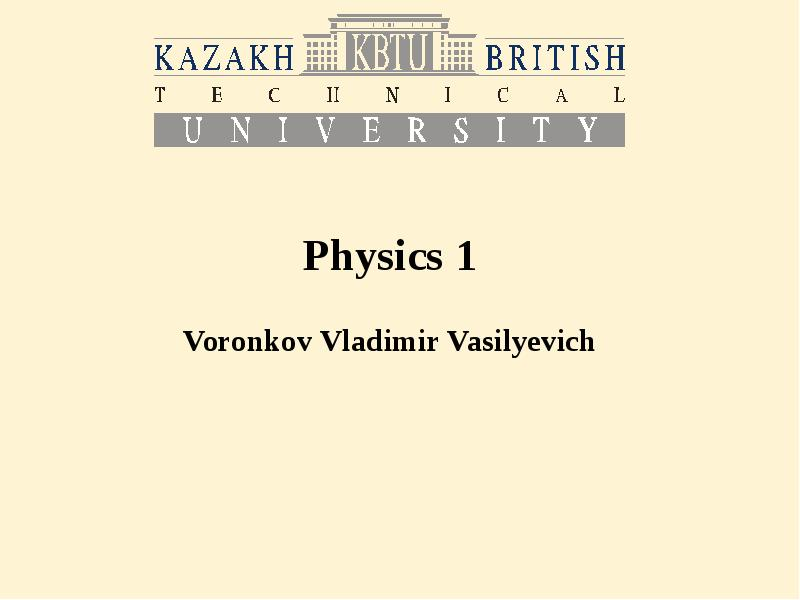Описание слайда: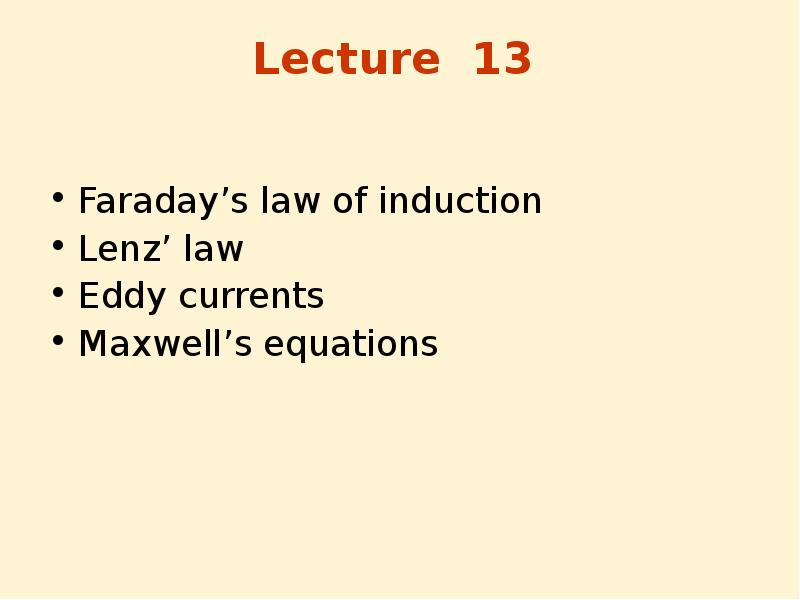Описание слайда:
Lecture 13 Faraday’s law of induction Lenz’ law Eddy currents Maxwell’s equations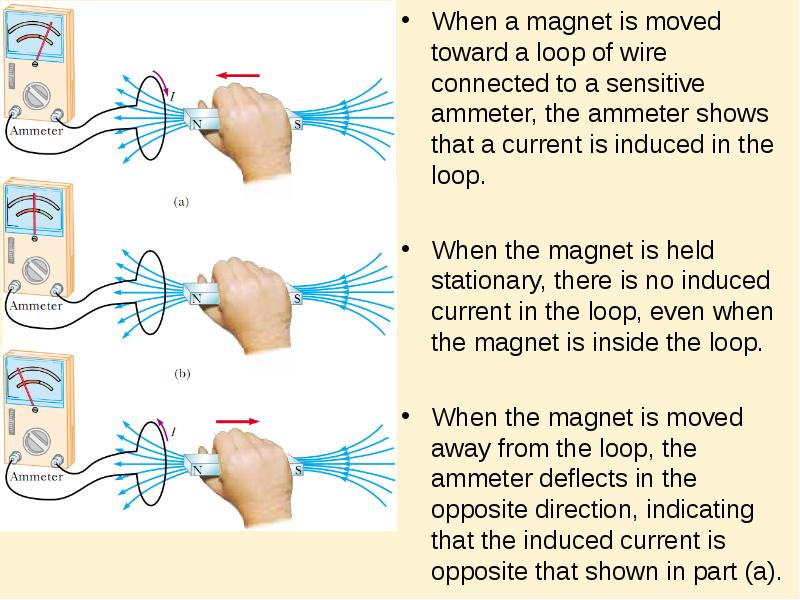Описание слайда:
When a magnet is moved toward a loop of wire connected to a sensitive ammeter, the ammeter shows that a current is induced in the loop. When a magnet is moved toward a loop of wire connected to a sensitive ammeter, the ammeter shows that a current is induced in the loop. When the magnet is held stationary, there is no induced current in the loop, even when the magnet is inside the loop. When the magnet is moved away from the loop, the ammeter deﬂects in the opposite direction, indicating that the induced current is opposite that shown in part (a).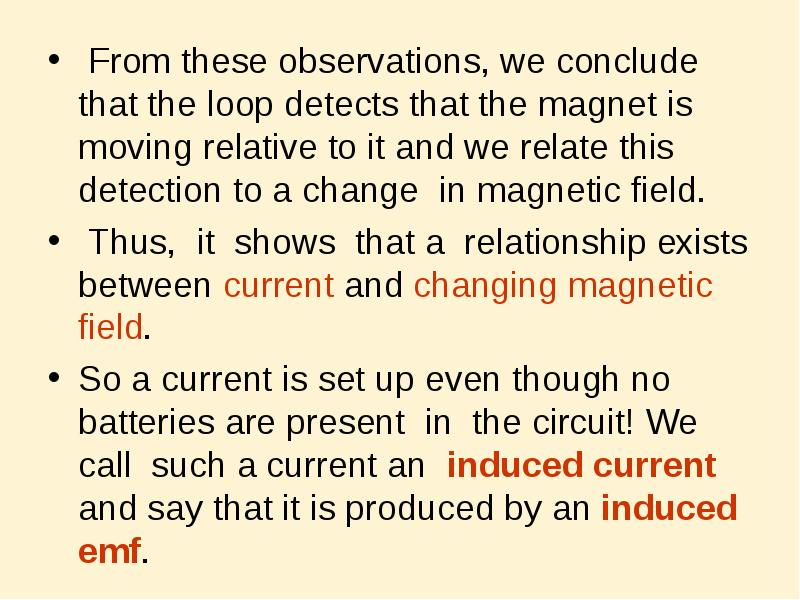Описание слайда:
From these observations, we conclude that the loop detects that the magnet is moving relative to it and we relate this detection to a change in magnetic ﬁeld. From these observations, we conclude that the loop detects that the magnet is moving relative to it and we relate this detection to a change in magnetic ﬁeld. Thus, it shows that a relationship exists between current and changing magnetic ﬁeld. So a current is set up even though no batteries are present in the circuit! We call such a current an induced current and say that it is produced by an induced emf.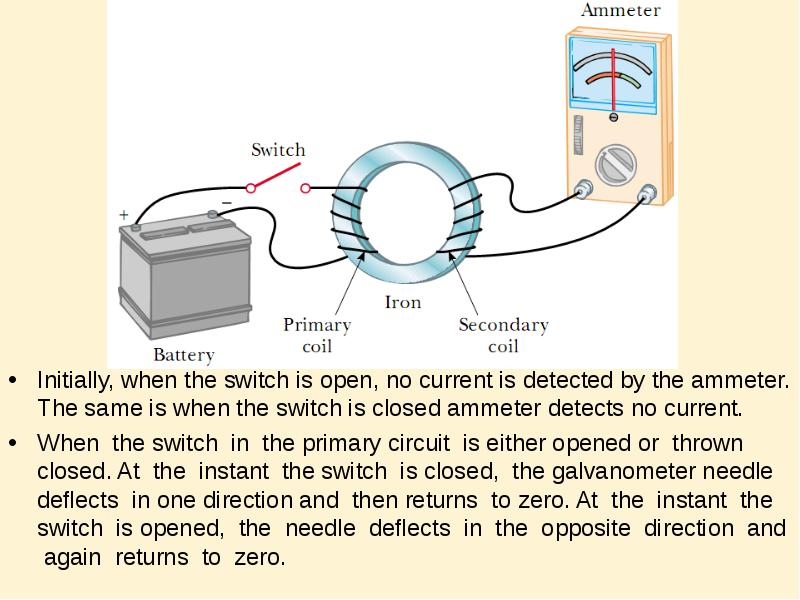Описание слайда:
Initially, when the switch is open, no current is detected by the ammeter. The same is when the switch is closed ammeter detects no current. Initially, when the switch is open, no current is detected by the ammeter. The same is when the switch is closed ammeter detects no current. When the switch in the primary circuit is either opened or thrown closed. At the instant the switch is closed, the galvanometer needle deﬂects in one direction and then returns to zero. At the instant the switch is opened, the needle deﬂects in the opposite direction and again returns to zero.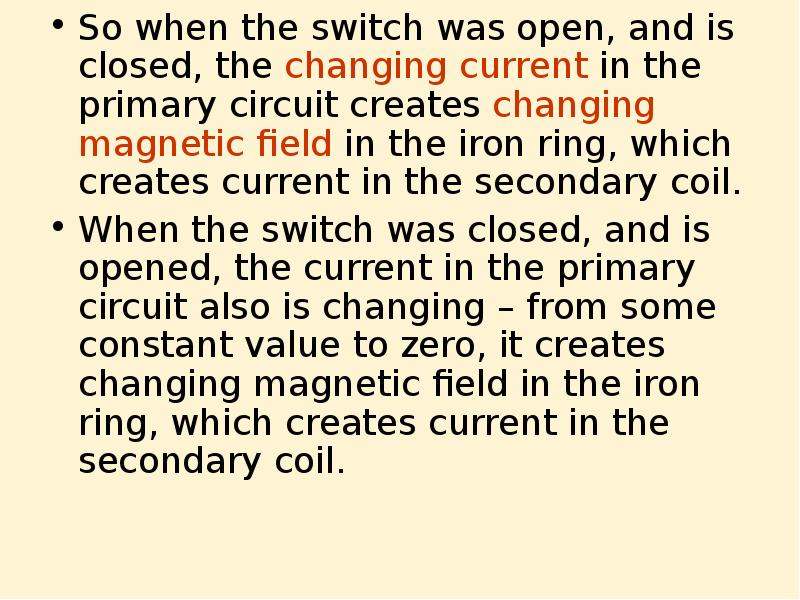Описание слайда:
So when the switch was open, and is closed, the changing current in the primary circuit creates changing magnetic field in the iron ring, which creates current in the secondary coil. So when the switch was open, and is closed, the changing current in the primary circuit creates changing magnetic field in the iron ring, which creates current in the secondary coil. When the switch was closed, and is opened, the current in the primary circuit also is changing – from some constant value to zero, it creates changing magnetic field in the iron ring, which creates current in the secondary coil.Описание слайда:
Summary of the two experiments An electric current can be induced in a circuit by a changing magnetic ﬁeld. The induced current exists only while the magnetic ﬁeld through the secondary coil is changing. Once the magnetic ﬁeld reaches a steady value, the current in the coil disappears.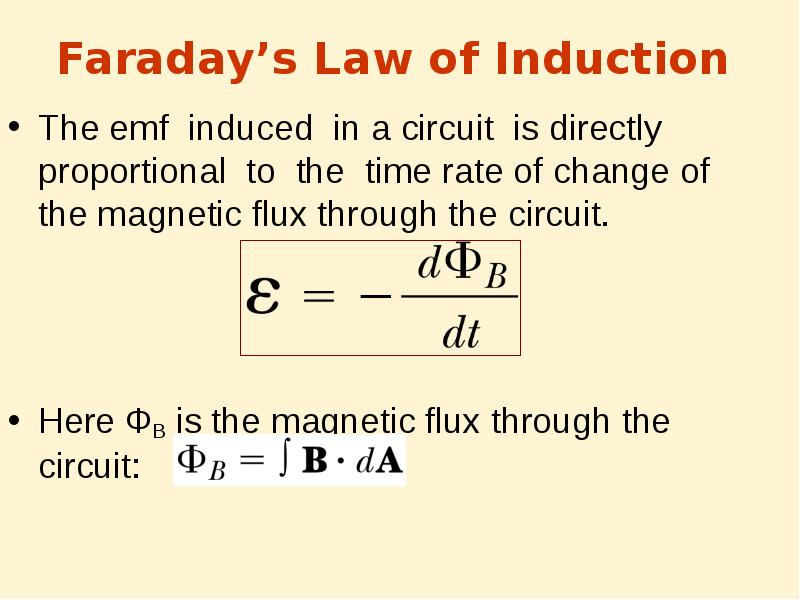Описание слайда:
Faraday’s Law of Induction The emf induced in a circuit is directly proportional to the time rate of change of the magnetic ﬂux through the circuit. Here ФB is the magnetic flux through the circuit: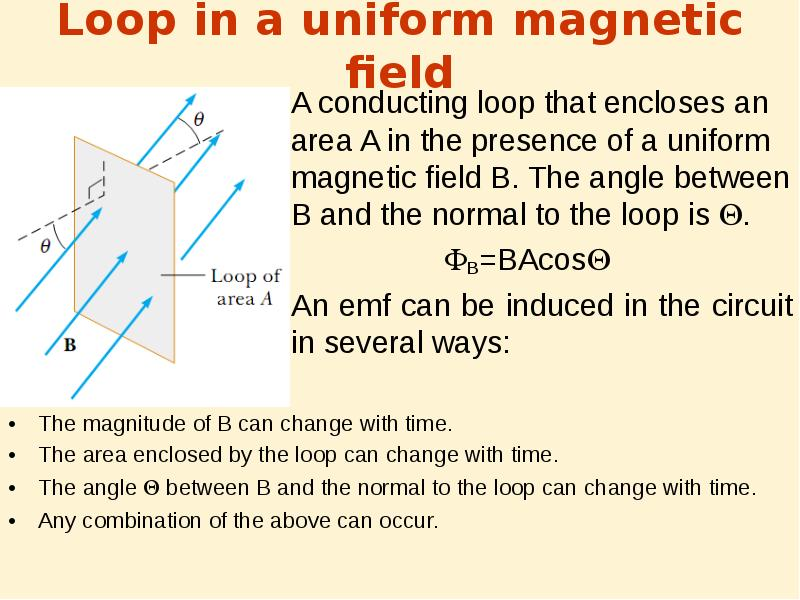Описание слайда:
Loop in a uniform magnetic field A conducting loop that encloses an area A in the presence of a uniform magnetic ﬁeld B. The angle between B and the normal to the loop is . B=BAcos An emf can be induced in the circuit in several ways: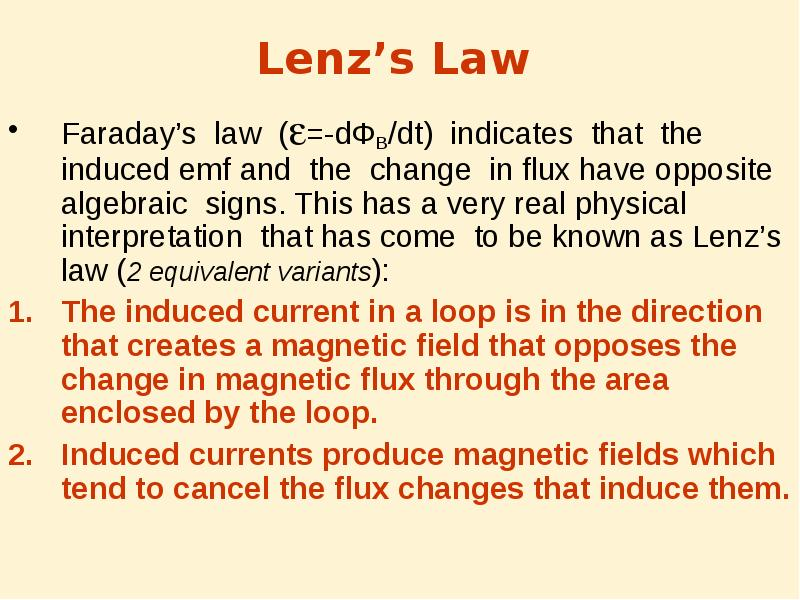Описание слайда:
Lenz’s Law Faraday’s law (=-dФB/dt) indicates that the induced emf and the change in ﬂux have opposite algebraic signs. This has a very real physical interpretation that has come to be known as Lenz’s law (2 equivalent variants): The induced current in a loop is in the direction that creates a magnetic ﬁeld that opposes the change in magnetic ﬂux through the area enclosed by the loop. Induced currents produce magnetic fields which tend to cancel the flux changes that induce them.Описание слайда:
Picture (a): When the magnet is moved toward the stationary conducting loop, a current is induced in the direction shown. The magnetic ﬁeld lines shown belong to the bar magnet. Picture (a): When the magnet is moved toward the stationary conducting loop, a current is induced in the direction shown. The magnetic ﬁeld lines shown belong to the bar magnet.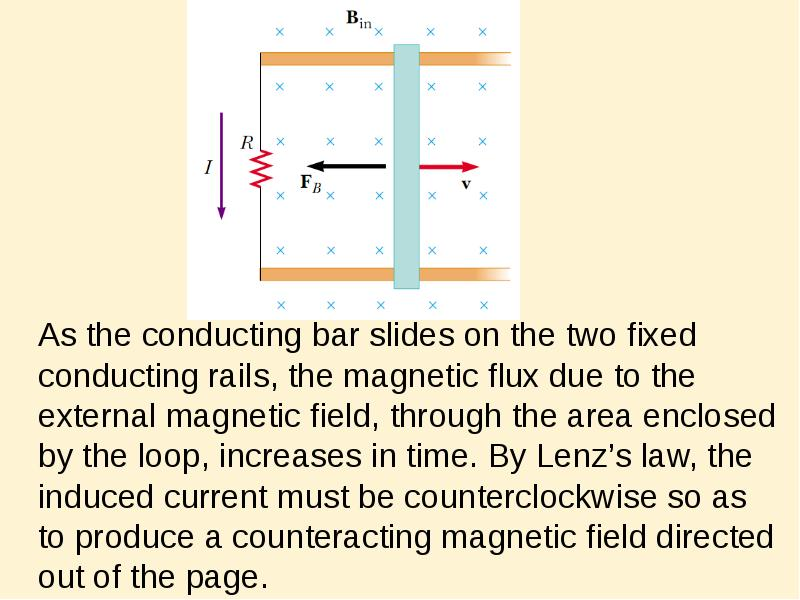Описание слайда:
As the conducting bar slides on the two ﬁxed conducting rails, the magnetic ﬂux due to the external magnetic ﬁeld, through the area enclosed by the loop, increases in time. By Lenz’s law, the induced current must be counterclockwise so as to produce a counteracting magnetic ﬁeld directed out of the page. As the conducting bar slides on the two ﬁxed conducting rails, the magnetic ﬂux due to the external magnetic ﬁeld, through the area enclosed by the loop, increases in time. By Lenz’s law, the induced current must be counterclockwise so as to produce a counteracting magnetic ﬁeld directed out of the page.Описание слайда:
Induced emf and Electric Fields A conducting loop of radius r in a uniform magnetic ﬁeld perpendicular to the plane of the loop. If B changes in time, an electric ﬁeld is induced in a direction tangent to the circumference of the loop.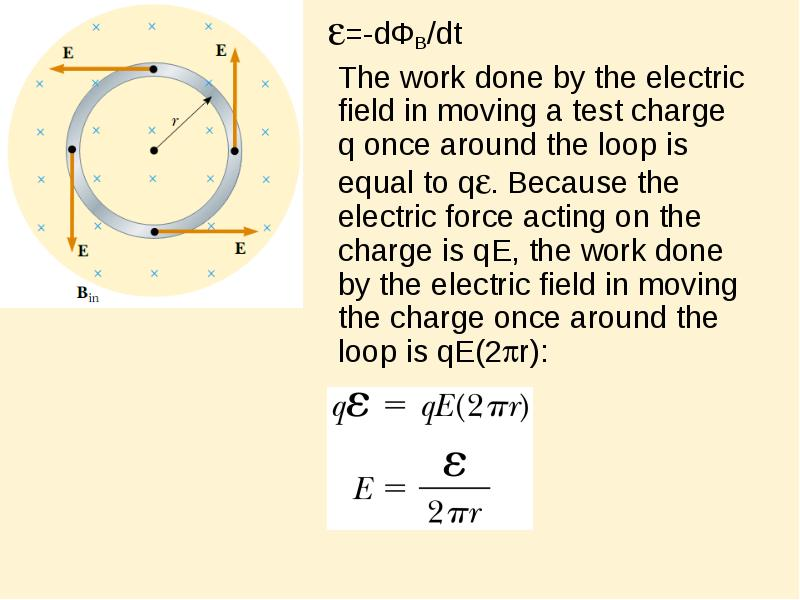Описание слайда:
=-dФB/dt =-dФB/dt The work done by the electric ﬁeld in moving a test charge q once around the loop is equal to q. Because the electric force acting on the charge is qE, the work done by the electric ﬁeld in moving the charge once around the loop is qE(2r):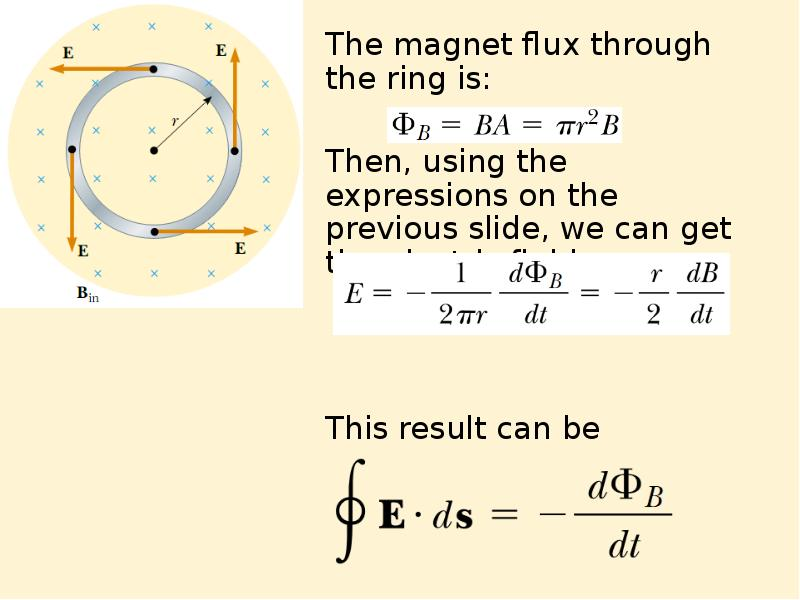Описание слайда:
The magnet flux through the ring is: The magnet flux through the ring is: Then, using the expressions on the previous slide, we can get the electric field: This result can be generalized: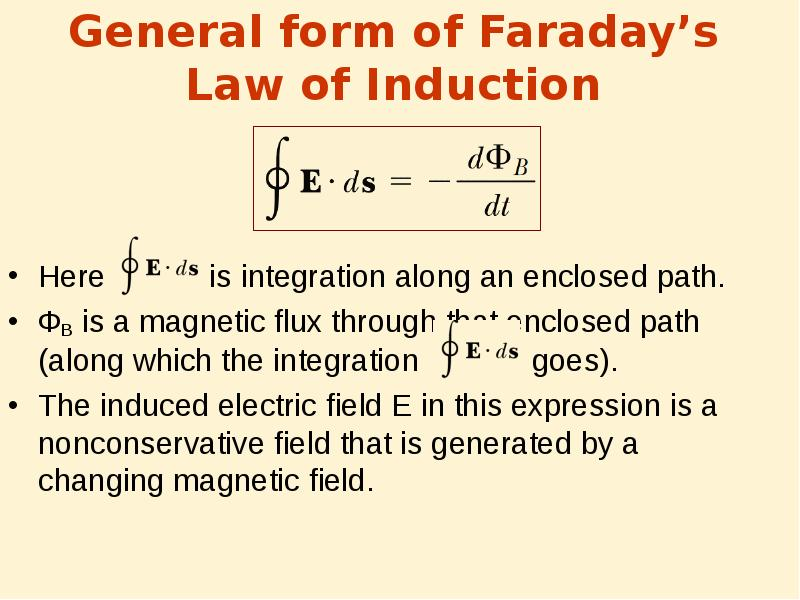Описание слайда:
General form of Faraday’s Law of Induction Here is integration along an enclosed path. ФB is a magnetic flux through that enclosed path (along which the integration goes). The induced electric ﬁeld E in this expression is a nonconservative ﬁeld that is generated by a changing magnetic ﬁeld.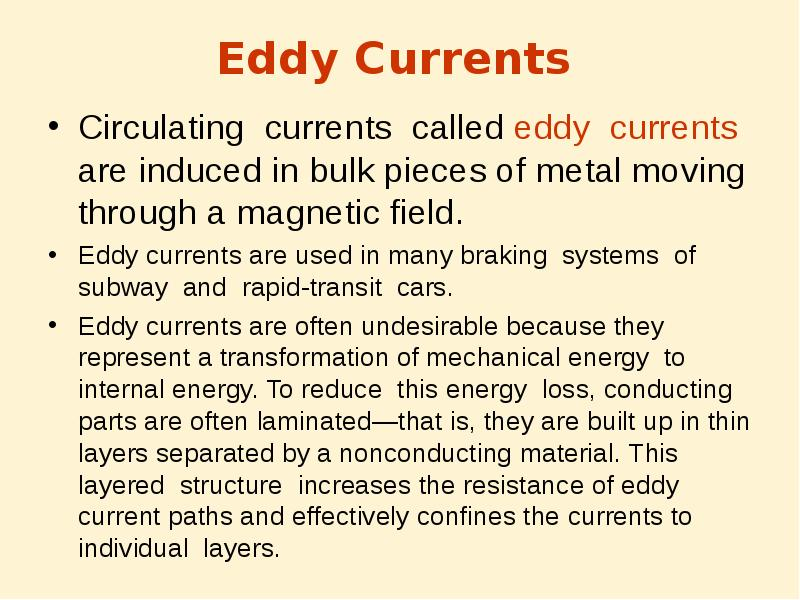Описание слайда:
Eddy Currents Circulating currents called eddy currents are induced in bulk pieces of metal moving through a magnetic ﬁeld. Eddy currents are used in many braking systems of subway and rapid-transit cars. Eddy currents are often undesirable because they represent a transformation of mechanical energy to internal energy. To reduce this energy loss, conducting parts are often laminated—that is, they are built up in thin layers separated by a nonconducting material. This layered structure increases the resistance of eddy current paths and effectively conﬁnes the currents to individual layers.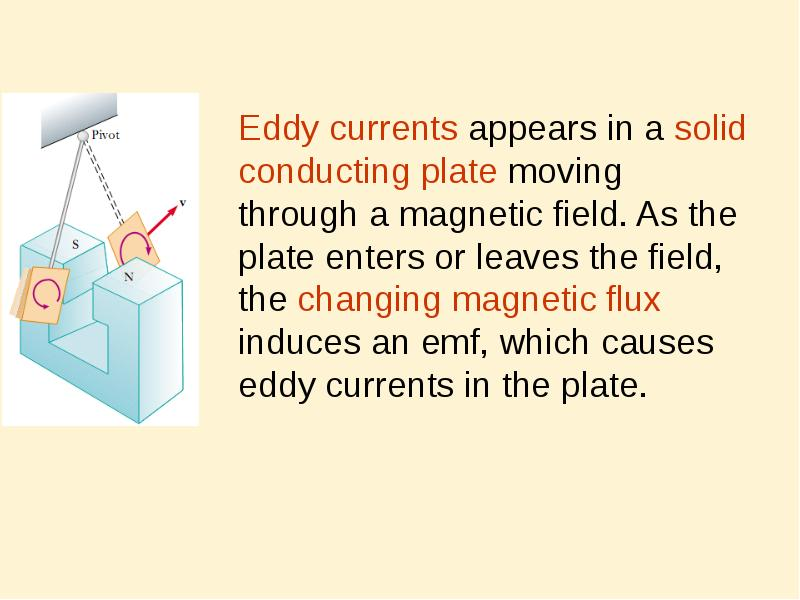Описание слайда:
Eddy currents appears in a solid conducting plate moving through a magnetic ﬁeld. As the plate enters or leaves the ﬁeld, the changing magnetic ﬂux induces an emf, which causes eddy currents in the plate. Eddy currents appears in a solid conducting plate moving through a magnetic ﬁeld. As the plate enters or leaves the ﬁeld, the changing magnetic ﬂux induces an emf, which causes eddy currents in the plate.Описание слайда:
As the conducting plate enters the ﬁeld (position 1), the eddy currents are counterclockwise. As the plate leaves the ﬁeld (position 2), the currents are clockwise. In either case, the force on the plate is opposite the velocity, and eventually the plate comes to rest. As the conducting plate enters the ﬁeld (position 1), the eddy currents are counterclockwise. As the plate leaves the ﬁeld (position 2), the currents are clockwise. In either case, the force on the plate is opposite the velocity, and eventually the plate comes to rest.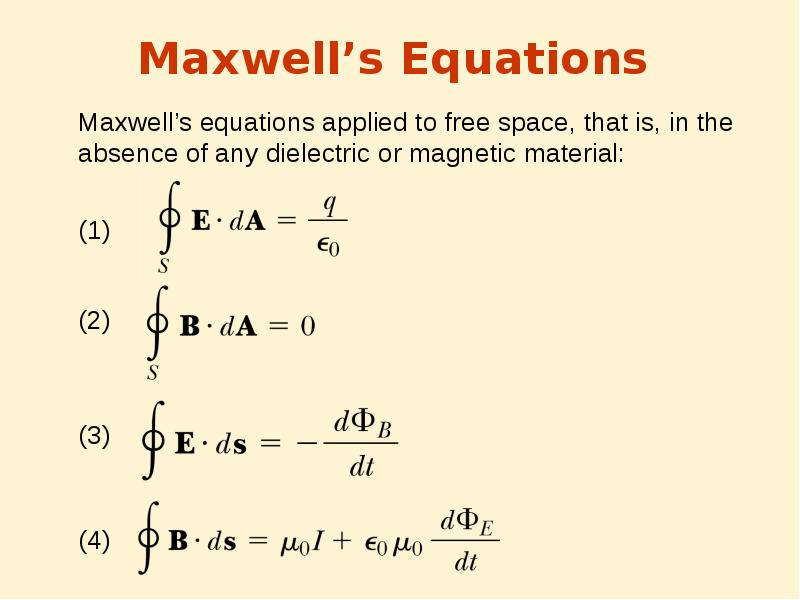Описание слайда:
Maxwell’s Equations Maxwell’s equations applied to free space, that is, in the absence of any dielectric or magnetic material: (1) (2) (3) (4)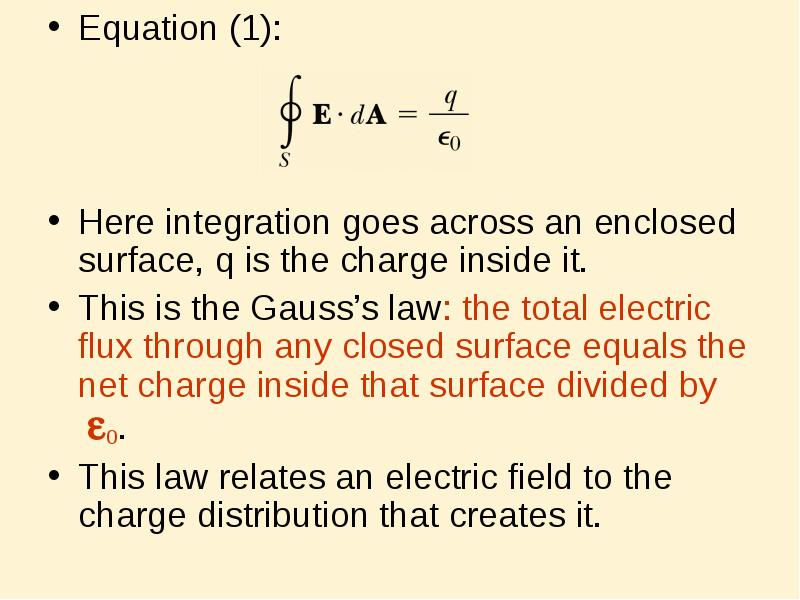Описание слайда:
Equation (1): Equation (1): Here integration goes across an enclosed surface, q is the charge inside it. This is the Gauss’s law: the total electric ﬂux through any closed surface equals the net charge inside that surface divided by 0. This law relates an electric ﬁeld to the charge distribution that creates it.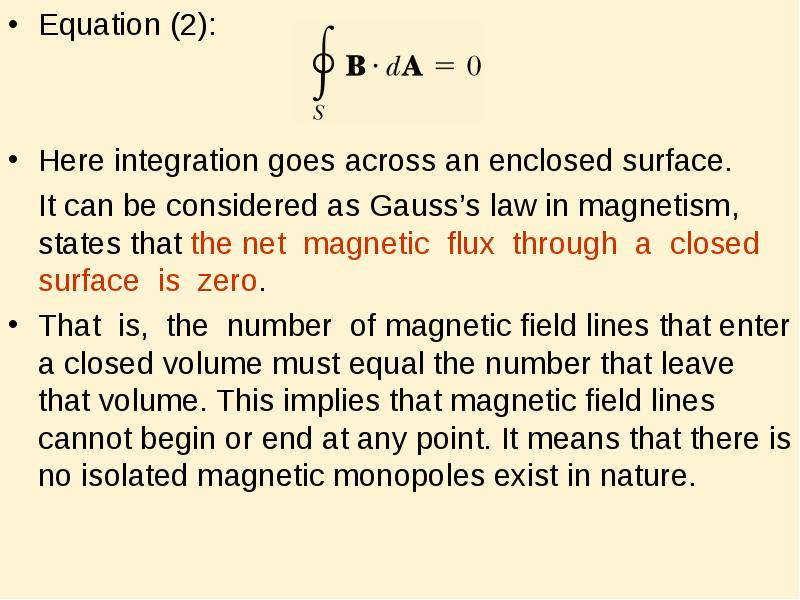Описание слайда:
Equation (2): Equation (2): Here integration goes across an enclosed surface. It can be considered as Gauss’s law in magnetism, states that the net magnetic ﬂux through a closed surface is zero. That is, the number of magnetic ﬁeld lines that enter a closed volume must equal the number that leave that volume. This implies that magnetic ﬁeld lines cannot begin or end at any point. It means that there is no isolated magnetic monopoles exist in nature.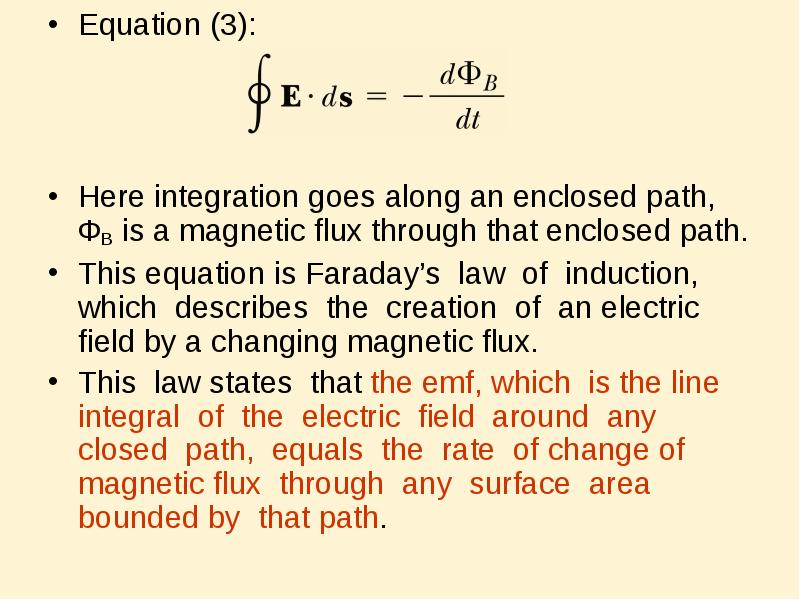Описание слайда:
Equation (3): Equation (3): Here integration goes along an enclosed path, ФB is a magnetic flux through that enclosed path. This equation is Faraday’s law of induction, which describes the creation of an electric ﬁeld by a changing magnetic ﬂux. This law states that the emf, which is the line integral of the electric ﬁeld around any closed path, equals the rate of change of magnetic ﬂux through any surface area bounded by that path.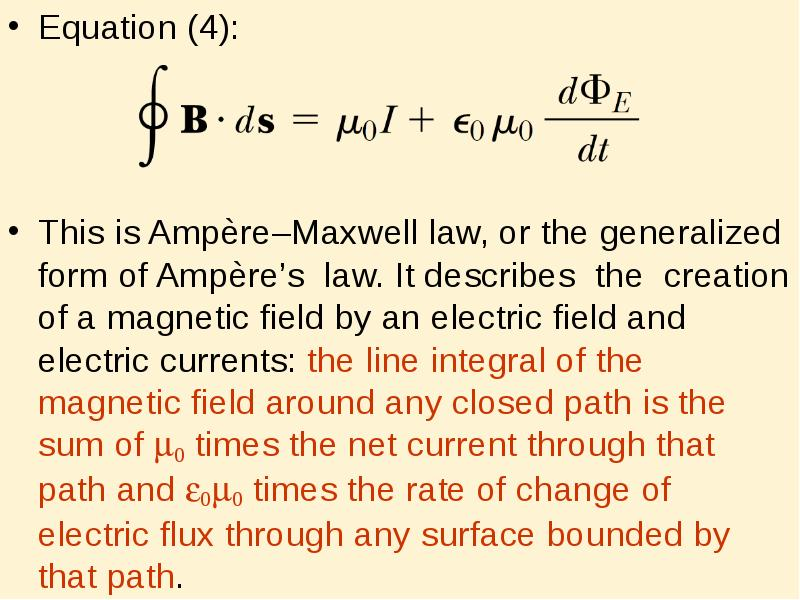Описание слайда:
Equation (4): Equation (4): This is Ampère–Maxwell law, or the generalized form of Ampère’s law. It describes the creation of a magnetic ﬁeld by an electric ﬁeld and electric currents: the line integral of the magnetic ﬁeld around any closed path is the sum of 0 times the net current through that path and 00 times the rate of change of electric ﬂux through any surface bounded by that path.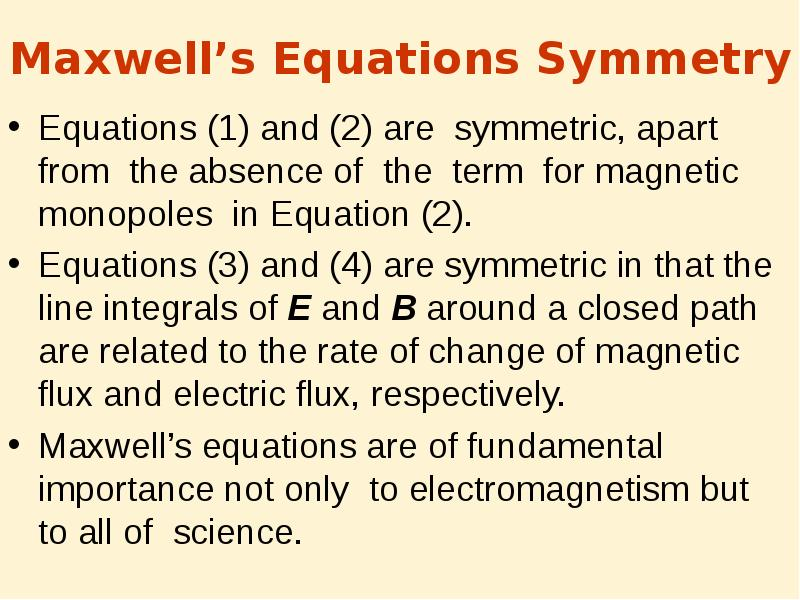Описание слайда:
Maxwell’s Equations Symmetry Equations (1) and (2) are symmetric, apart from the absence of the term for magnetic monopoles in Equation (2). Equations (3) and (4) are symmetric in that the line integrals of E and B around a closed path are related to the rate of change of magnetic ﬂux and electric ﬂux, respectively. Maxwell’s equations are of fundamental importance not only to electromagnetism but to all of science.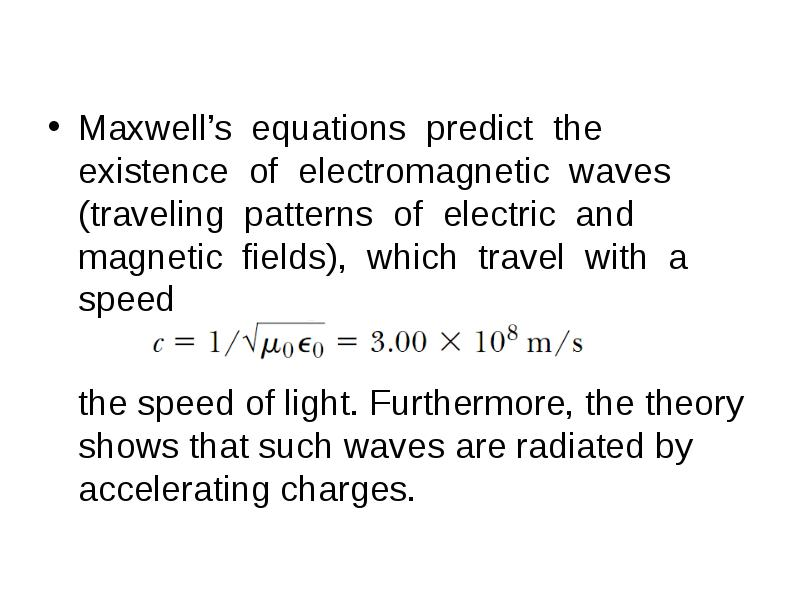Описание слайда:
Maxwell’s equations predict the existence of electromagnetic waves (traveling patterns of electric and magnetic ﬁelds), which travel with a speed Maxwell’s equations predict the existence of electromagnetic waves (traveling patterns of electric and magnetic ﬁelds), which travel with a speed the speed of light. Furthermore, the theory shows that such waves are radiated by accelerating charges.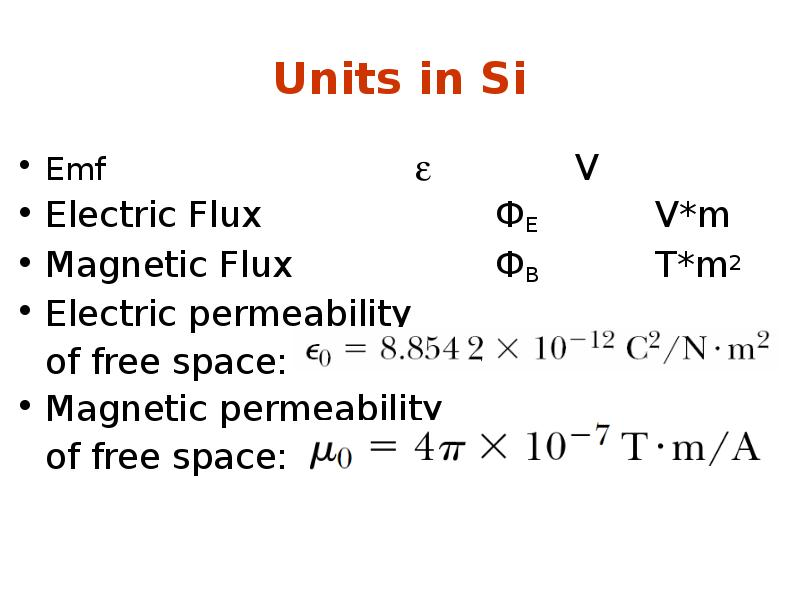Описание слайда:
Units in Si Emf  V Electric Flux ФE V*m Magnetic Flux ФB T*m2 Electric permeability of free space: Magnetic permeability of free space:

Скачать презентацию на тему Faraday’s law of induction/ можно ниже:

Похожие презентации